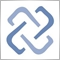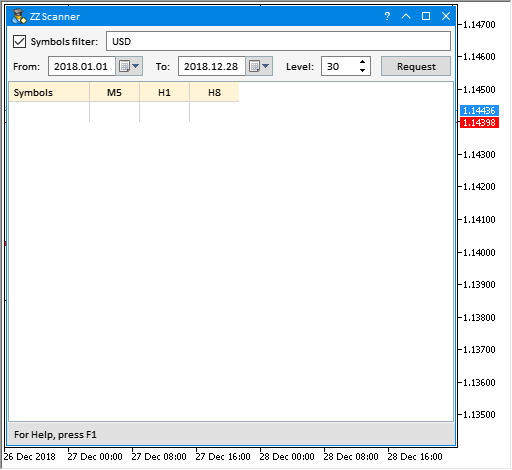# Discussion of article "The power of ZigZag (part II). Examples of receiving, processing and displaying data"Moderator
222238

New article The power of ZigZag (part II). Examples of receiving, processing and displaying data has been published:

In the first part of the article, I have described a modified ZigZag indicator and a class for receiving data of that type of indicators. Here, I will show how to develop indicators based on these tools and write an EA for tests that features making deals according to signals formed by ZigZag indicator. As an addition, the article will introduce a new version of the EasyAndFast library for developing graphical user interfaces.

Below you can see how this looks in the GUI. In this case, the results show that the lesser the value, the lesser the number of trends in the considered area. It would be wise to set as wide range of dates as possible in order to obtain information using as much data as possible.Fig. 12. Color scale for visualizing table data

The wider the range of dates, the more data are used and, accordingly, the more time it will take to generate data and calculate the parameters. If there is not enough data, an attempt is made to download them from the server.

Author: Anatoli Kazharski1234

ANatoli, Nice work!

One question ...

The MultiPercentageSegmentZZ looks like it has a bug in the attached files. GetZigZagData function of zz_current0, zz_current1, zz_current2 does not have h_buff,l_buff

Should it be like this?

```//+------------------------------------------------------------------+
//| Fill in the indicator buffers                                    |
//+------------------------------------------------------------------+
void FillIndicatorBuffers(const int i,const int total,const datetime &time[])
{
int index=total-i-1;
int copy_total=1000;
int h_buff=2,l_buff=3;
datetime start_time_in =NULL;
datetime stop_time_in  =NULL;
//--- Get source data from a higher timeframe
datetime stop_time=time[i]-(PeriodSeconds(HigherTimeframe)*copy_total);
CopyBuffer(zz_handle_htf,2,time[i],stop_time,h_zz_buffer_temp);
CopyBuffer(zz_handle_htf,3,time[i],stop_time,l_zz_buffer_temp);
CopyTime(_Symbol,HigherTimeframe,time[i],stop_time,t_zz_buffer_temp);
//--- Get final data from a higher timeframe
zz_higher_tf.GetZigZagData(h_zz_buffer_temp,l_zz_buffer_temp,t_zz_buffer_temp);
double htf_value=zz_higher_tf.PercentSumSegmentsDifference();
//--- First segment data
zz_higher_tf.SegmentTimes(zz_handle_current,h_buff,l_buff,_Symbol,HigherTimeframe,_Period,0,start_time_in,stop_time_in);
zz_current0.GetZigZagData(zz_handle_current,h_buff,l_buff,_Symbol,_Period,start_time_in,stop_time_in);
//--- Second segment data
zz_higher_tf.SegmentTimes(zz_handle_current,h_buff,l_buff,_Symbol,HigherTimeframe,_Period,1,start_time_in,stop_time_in);
zz_current1.GetZigZagData(zz_handle_current,h_buff,l_buff,_Symbol,_Period,start_time_in,stop_time_in);
//--- Third segment data
zz_higher_tf.SegmentTimes(zz_handle_current,h_buff,l_buff,_Symbol,HigherTimeframe,_Period,2,start_time_in,stop_time_in);
zz_current2.GetZigZagData(zz_handle_current,h_buff,l_buff,_Symbol,_Period,start_time_in,stop_time_in);
//--- On the last bar
if(i<total-1)
{
buffer_zz_higher_tf[i] =htf_value;
buffer_segment_0[i]    =zz_current0.PercentSumSegmentsDifference();
buffer_segment_1[i]    =zz_current1.PercentSumSegmentsDifference();
buffer_segment_2[i]    =zz_current2.PercentSumSegmentsDifference();
}
//--- On history
else
{
//--- In case there is a new bar of the higher timeframe
if(new_bar_time!=t_zz_buffer_temp)
{
new_bar_time=t_zz_buffer_temp;
//---
if(i>2)
{
int f=1,s=2;
buffer_zz_higher_tf[i-f] =buffer_zz_higher_tf[i-s];
buffer_segment_0[i-f]    =buffer_segment_0[i-s];
buffer_segment_1[i-f]    =buffer_segment_1[i-s];
buffer_segment_2[i-f]    =buffer_segment_2[i-s];
}
}
else
{
buffer_zz_higher_tf[i] =htf_value;
buffer_segment_0[i]    =zz_current0.PercentSumSegmentsDifference();
buffer_segment_1[i]    =zz_current1.PercentSumSegmentsDifference();
buffer_segment_2[i]    =zz_current2.PercentSumSegmentsDifference();
}
}
}
//+------------------------------------------------------------------+
```